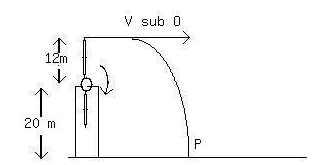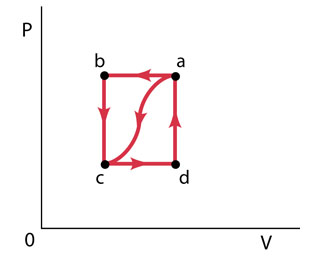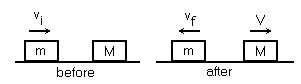Ask question

# A wind farm generator uses a two-bladed propellermounted on a pylon at a height of 20 m. The length of eachpropeller blade is 12 m. A tip of the propeller breaks offwhen the propeller is vertical. The fragment flies offhorizontally, falls, and strikes the ground at P. Just beforethe fragment broke off, the propeller was turning uniformly, taking1.2 s for each rotation. In the above figure, the distancefrom the base of the pylon to the point where the fragment strikesthe ground is closest to: a) 130 m b) 160 m c) 120 m d) 140 m e) 150 m# A wind farm generator uses a two-bladed propellermounted on a pylon at a height of 20 m. The length of eachpropeller blade is 12 m. A tip of the propeller breaks offwhen the propeller is vertical. The fragment flies offhorizontally, falls, and strikes the ground at P. Just beforethe fragment broke off, the propeller was turning uniformly, taking1.2 s for each rotation. In the above figure, the distancefrom the base of the pylon to the point where the fragment strikesthe ground is closest to: a) 130 m b) 160 m c) 120 m d) 140 m e) 150 m

Question
Otherasked 2021-04-24A wind farm generator uses a two-bladed propellermounted on a pylon at a height of 20 m. The length of eachpropeller blade is 12 m. A tip of the propeller breaks offwhen the propeller is vertical. The fragment flies offhorizontally, falls, and strikes the ground at P. Just beforethe fragment broke off, the propeller was turning uniformly, taking1.2 s for each rotation. In the above figure, the distancefrom the base of the pylon to the point where the fragment strikesthe ground is closest to:
a) 130 m
b) 160 m
c) 120 m
d) 140 m
e) 150 m

## Answers (1)2021-04-26
To find the velocity of the propeller:
find the cricumference of the propeller blade:
$$\displaystyle\pi{r}^{{{2}}}=\pi{\left({12}^{{{2}}}\right)}={144}\pi$$
if it travels this distance in 1.2 seconds:
$$\displaystyle{v}={144}\frac{\pi}{{1.2}}={120}\pi\frac{{m}}{{s}}$$
The total height is 32 m, so use equation of motion todetermine the time of flight:
d = 32 m
$$\displaystyle{a}\frac{=}{{9.8}}\frac{{m}}{{s}^{{{2}}}}$$
t = ?
$$\displaystyle{V}_{{{i}}}={0}$$
$$\displaystyle{d}={V}{t}+\frac{{1}}{{2}}{a}{t}^{{{2}}}={2.55}$$ seconds
now put this into $$\displaystyle{V}_{{{o}}}=\frac{{x}}{{t}}$$ and solve for x:
$$\displaystyle\frac{{V}_{{{o}}}}{{t}}={x}={120}\frac{\pi}{{2.55}}={147.8}{m}$$
so this would correspond to e).

### Relevant Questionsasked 2021-05-06
A spring is mounted vertically on the floor. The mass of the spring is negligible. A certain object is placed on the spring to compress it. When the object is pushed further down by just a bit and then released, one up/down oscillation cycle occurs in 0.250 s. However, when the object is pushed down by 5.00 X 10^-2 m to point P and then released, the object flies entirely off the spring. To what height above point P does the object rise in the absence of air resistance?asked 2021-03-26
A helicopter carrying dr. evil takes off with a constant upward acceleration of $$\displaystyle{5.0}\ \frac{{m}}{{s}^{{2}}}$$. Secret agent austin powers jumps on just as the helicopter lifts off the ground. Afterthe two men struggle for 10.0 s, powers shuts off the engineand steps out of the helicopter. Assume that the helicopter is infree fall after its engine is shut off and ignore effects of airresistance.
a) What is the max height above ground reached by the helicopter?
b) Powers deploys a jet pack strapped on his back 7.0s after leaving the helicopter, and then he has a constant downward acceleration with magnitude 2.0 m/s2. how far is powers above the ground when the helicopter crashes into the ground.asked 2021-05-04When a gas is taken from a to c along the curved path in the figure (Figure 1) , the work done by the gas is W = -40 J and the heat added to the gas is Q = -140 J . Along path abc, the work done by the gas is W = -50 J . (That is, 50 J of work is done on the gas.)
I keep on missing Part D. The answer for part D is not -150,150,-155,108,105( was close but it said not quite check calculations)
Part A
What is Q for path abc?
Express your answer to two significant figures and include the appropriate units.
Part B
f Pc=1/2Pb, what is W for path cda?
Express your answer to two significant figures and include the appropriate units.
Part C
What is Q for path cda?
Express your answer to two significant figures and include the appropriate units.
Part D
What is Ua?Uc?
Express your answer to two significant figures and include the appropriate units.
Part E
If Ud?Uc=42J, what is Q for path da?
Express your answer to two significant figures and include the appropriate units.asked 2021-02-23A block of mass m=3.6 kg, moving on africtionless surface with a speed $$\displaystyle{v}_{{1}}={9.3}$$ m/s makes a perfectly elastic collision with a block of mass Mat rest. After the collision, the 3.6 kg block recoils with a speed of $$\displaystyle{v}_{{1}}={2.7}$$ m/s in figure, the speed of the vlock of mass M after the collision is closest to:
a. 9.3 m/s
b. 6.6 m/s
c. 8.0 m/s
d. 10.7 m/s
e. 12.0 m/sasked 2021-03-12
A 75.0-kg man steps off a platform 3.10 m above the ground. Hekeeps his legs straight as he falls, but at the moment his feettouch the ground his knees begin to bend, and, treated as aparticle, he moves an additional 0.60 m before coming torest.
a) what is the speed at the instant his feet touch theground?
b) treating him as a particle, what is his acceleration(magnitude and direction) as he slows down, if the acceleration isassumed to be constant?
c) draw his free-body diagram (see section 4.6). in termsof forces on the diagram, what is the net force on him? usenewton's laws and the results of part (b) to calculate the averageforce his feet exert on the ground while he slows down. expressthis force in newtons and also as a multiple of his weight.asked 2021-02-19
An airplane propeller is 2.08 m in length (from tip to tip) and has a mass of 117 kg. When the airpline's engine is first started, it applies a constant torque of $$\displaystyle{1950}\ {N}\cdot{m}$$ to the propeller, which starts from rest.
a) What is the angular acceleration of the propeller? Model the propeller as a slender rod.
b) What is the propeller's angular speed after making 5.00 revolutions?
c) How much work is done by the engine during the first 5.00 revolutions?
e) What is the instantaneous power output of the motor at the instant that the propeller has turne through 5.00 revolutions?asked 2021-04-11
The equation F=−vex(dm/dt) for the thrust on a rocket, can also be applied to an airplane propeller. In fact, there are two contributions to the thrust: one positive and one negative. The positive contribution comes from air pushed backward, away from the propeller (so dm/dt<0), at a speed vex relative to the propeller. The negative contribution comes from this same quantity of air flowing into the front of the propeller (so dm/dt>0) at speed v, equal to the speed of the airplane through the air.
For a Cessna 182 (a single-engine airplane) flying at 130 km/h, 150 kg of air flows through the propeller each second and the propeller develops a net thrust of 1300 N. Determine the speed increase (in km/h) that the propeller imparts to the air.asked 2021-04-25
The unstable nucleus uranium-236 can be regarded as auniformly charged sphere of charge Q=+92e and radius $$\displaystyle{R}={7.4}\times{10}^{{-{15}}}$$ m. In nuclear fission, this can divide into twosmaller nuclei, each of 1/2 the charge and 1/2 the voume of theoriginal uranium-236 nucleus. This is one of the reactionsthat occurred n the nuclear weapon that exploded over Hiroshima, Japan in August 1945.
A. Find the radii of the two "daughter" nuclei of charge+46e.
B. In a simple model for the fission process, immediatelyafter the uranium-236 nucleus has undergone fission the "daughter"nuclei are at rest and just touching. Calculate the kineticenergy that each of the "daughter" nuclei will have when they arevery far apart.
C. In this model the sum of the kinetic energies of the two"daughter" nuclei is the energy released by the fission of oneuranium-236 nucleus. Calculate the energy released by thefission of 10.0 kg of uranium-236. The atomic mass ofuranium-236 is 236 u, where 1 u = 1 atomic mass unit $$\displaystyle={1.66}\times{10}^{{-{27}}}$$ kg. Express your answer both in joules and in kilotonsof TNT (1 kiloton of TNT releases 4.18 x 10^12 J when itexplodes).asked 2021-05-18
The student engineer of a campus radio station wishes to verify the effectivencess of the lightning rod on the antenna mast. The unknown resistance $$\displaystyle{R}_{{x}}$$ is between points C and E. Point E is a "true ground", but is inaccessible for direct measurement because the stratum in which it is located is several meters below Earth's surface. Two identical rods are driven into the ground at A and B, introducing an unknown resistance $$\displaystyle{R}_{{y}}$$. The procedure for finding the unknown resistance $$\displaystyle{R}_{{x}}$$ is as follows. Measure resistance $$\displaystyle{R}_{{1}}$$ between points A and B. Then connect A and B with a heavy conducting wire and measure resistance $$\displaystyle{R}_{{2}}$$ between points A and C.Derive a formula for $$\displaystyle{R}_{{x}}$$ in terms of the observable resistances $$\displaystyle{R}_{{1}}$$ and $$\displaystyle{R}_{{2}}$$. A satisfactory ground resistance would be $$\displaystyle{R}_{{x}}{<}{2.0}$$ Ohms. Is the grounding of the station adequate if measurments give $$\displaystyle{R}_{{1}}={13}{O}{h}{m}{s}$$ and R_2=6.0 Ohms?asked 2021-05-16
Consider the curves in the first quadrant that have equationsy=Aexp(7x), where A is a positive constant. Different valuesof A give different curves. The curves form a family,F. Let P=(6,6). Let C be the number of the family Fthat goes through P.
A. Let y=f(x) be the equation of C. Find f(x).
B. Find the slope at P of the tangent to C.
C. A curve D is a perpendicular to C at P. What is the slope of thetangent to D at the point P?
D. Give a formula g(y) for the slope at (x,y) of the member of Fthat goes through (x,y). The formula should not involve A orx.
E. A curve which at each of its points is perpendicular to themember of the family F that goes through that point is called anorthogonal trajectory of F. Each orthogonal trajectory to Fsatisfies the differential equation dy/dx = -1/g(y), where g(y) isthe answer to part D.
Find a function of h(y) such that x=h(y) is the equation of theorthogonal trajectory to F that passes through the point P.
...